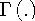Functions and CALL Routines

# BETA Function

Returns the value of the beta function.
 Category: Mathematical

## Syntax

 BETA(a,b)

### Arguments

a

is the first shape parameter, where a>0.

b

is the second shape parameter, where b>0.

The BETA function is mathematically given by the equationwith a>0, b>0. It should be noted thatwhereis the gamma function.

If the expression cannot be computed, BETA returns a missing value.

SAS Statements Results
`x=beta(5,3);`
`0.9523809524e-2`

 Function:Previous Page | Next Page | Top of Page